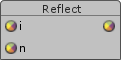## FANDOM

167 Pages## NameEdit

Reflect - returns the reflectiton vector given an incidence vector and a normal vector.

## SynopsisEdit

```vector1 reflect(vector1 i, vector1 n);
vector2 reflect(vector2 i, vector2 n);
vector3 reflect(vector3 i, vector3 n);
vector4 reflect(vector4 i, vector4 n);
```

## ParametersEdit

i
Incidence vector.
n
Normal vector.

## DescriptionEdit

Returns the reflectiton vector given an incidence vector i and a normal vector n. The resulting vector is the identical number of components as the two input vectors.

The normal vector n should be normalized. If n is normalized, the output vector will have the same length as the input incidence vector i.